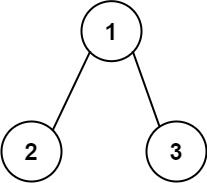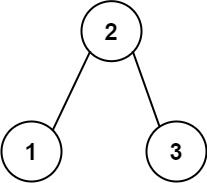##### Welcome to Subscribe On Youtube

Formatted question description: https://leetcode.ca/all/549.html

# 549. Binary Tree Longest Consecutive Sequence II

## Description

Given the root of a binary tree, return the length of the longest consecutive path in the tree.

A consecutive path is a path where the values of the consecutive nodes in the path differ by one. This path can be either increasing or decreasing.

• For example, [1,2,3,4] and [4,3,2,1] are both considered valid, but the path [1,2,4,3] is not valid.

On the other hand, the path can be in the child-Parent-child order, where not necessarily be parent-child order.

Example 1:Input: root = [1,2,3]
Output: 2
Explanation: The longest consecutive path is [1, 2] or [2, 1].


Example 2:Input: root = [2,1,3]
Output: 3
Explanation: The longest consecutive path is [1, 2, 3] or [3, 2, 1].


Constraints:

• The number of nodes in the tree is in the range [1, 3 * 104].
• -3 * 104 <= Node.val <= 3 * 104

## Solutions

• 
public class Binary_Tree_Longest_Consecutive_Sequence_II {

class Solution {
public int longestConsecutive(TreeNode root) {

if (root == null) {
return 0;
}

int res = helper(root, 1) + helper(root, -1) + 1; // +1 is increasing, -1 is decreasing
return Math.max(res, Math.max(longestConsecutive(root.left), longestConsecutive(root.right)));
}

int helper(TreeNode node, int diff) {
if (node == null) {
return 0;
}

int left = 0, right = 0;
if (node.left != null && node.val - node.left.val == diff) {
left = 1 + helper(node.left, diff);
}
if (node.right != null && node.val - node.right.val == diff) {
right = 1 + helper(node.right, diff);
}

return Math.max(left, right);
}
}
}

############

/**
* Definition for a binary tree node.
* public class TreeNode {
*     int val;
*     TreeNode left;
*     TreeNode right;
*     TreeNode() {}
*     TreeNode(int val) { this.val = val; }
*     TreeNode(int val, TreeNode left, TreeNode right) {
*         this.val = val;
*         this.left = left;
*         this.right = right;
*     }
* }
*/
class Solution {
private int ans;

public int longestConsecutive(TreeNode root) {
ans = 0;
dfs(root);
return ans;
}

private int[] dfs(TreeNode root) {
if (root == null) {
return new int[] {0, 0};
}
int incr = 1, decr = 1;
int[] left = dfs(root.left);
int[] right = dfs(root.right);
if (root.left != null) {
if (root.left.val + 1 == root.val) {
incr = left + 1;
}
if (root.left.val - 1 == root.val) {
decr = left + 1;
}
}
if (root.right != null) {
if (root.right.val + 1 == root.val) {
incr = Math.max(incr, right + 1);
}
if (root.right.val - 1 == root.val) {
decr = Math.max(decr, right + 1);
}
}
ans = Math.max(ans, incr + decr - 1);
return new int[] {incr, decr};
}
}

• // OJ: https://leetcode.com/problems/binary-tree-longest-consecutive-sequence-ii/
// Time: O(N)
// Space: O(H)
class Solution {
int ans = 0;
pair<int, int> dfs(TreeNode *root) {
if (!root) return {0, 0};
auto [linc, ldec] = dfs(root->left);
auto [rinc, rdec] = dfs(root->right);
if (root->left) {
if (root->val != root->left->val + 1) linc = 0;
if (root->val != root->left->val - 1) ldec = 0;
}
if (root->right) {
if (root->val != root->right->val + 1) rinc = 0;
if (root->val != root->right->val - 1) rdec = 0;
}
ans = max({ ans, ldec + rinc + 1, rdec + linc + 1 });
return { max(linc, rinc) + 1, max(ldec, rdec) + 1 };
}
public:
int longestConsecutive(TreeNode* root) {
dfs(root);
return ans;
}
};

• # Definition for a binary tree node.
# class TreeNode(object):
#     def __init__(self, x):
#         self.val = x
#         self.left = None
#         self.right = None

'''
>>> float('-inf')+1
-inf
>>> float('-inf')-1
-inf
'''
class Solution(object):
def longestConsecutive(self, root):
"""
:type root: TreeNode
:rtype: int
"""

def dfs(root):
if not root:
# val, increasing length, decreasing length, max length
return float('-inf'), 0, 0, 0
# inc/dec starting from root
inc = dec = 1
left, leftInc, leftDec, leftMax = dfs(root.left)
right, rightInc, rightDec, rightMax = dfs(root.right)
if root.val + 1 == left:
inc = max(leftInc + 1, inc)
if root.val - 1 == left:
dec = max(leftDec + 1, dec)
if root.val + 1 == right:
inc = max(rightInc + 1, inc)
if root.val - 1 == right:
dec = max(rightDec + 1, dec)
# note: max may occur in children subtree, not current root's tree
return root.val, inc, dec, max(inc + dec - 1, leftMax, rightMax, inc,
dec)  # -1 because root is counted twice, even left/right both null

return dfs(root)

############

# Definition for a binary tree node.
# class TreeNode:
#     def __init__(self, val=0, left=None, right=None):
#         self.val = val
#         self.left = left
#         self.right = right
class Solution:
def longestConsecutive(self, root: TreeNode) -> int:
def dfs(root):
if root is None:
return [0, 0]
nonlocal ans
incr = decr = 1
i1, d1 = dfs(root.left)
i2, d2 = dfs(root.right)
if root.left:
if root.left.val + 1 == root.val:
incr = i1 + 1
if root.left.val - 1 == root.val:
decr = d1 + 1
if root.right:
if root.right.val + 1 == root.val:
incr = max(incr, i2 + 1) # incr1 and incr2 must be in different tree branches, eg. left child cannot be both increasing and decreasing. Same as decr1 and decr2
if root.right.val - 1 == root.val:
decr = max(decr, d2 + 1)
ans = max(ans, incr + decr - 1) # -1 because root is counted twice, even left/right both null
return [incr, decr]

ans = 0
dfs(root)
return ans

class Solution: # issue with this solution, duplicated dfs() search
def longestConsecutive(self, root: TreeNode) -> int:
def helper(node: TreeNode, diff: int) -> int:
if not node:
return 0

left = right = 0
if node.left and node.val - node.left.val == diff:
left = 1 + helper(node.left, diff)
if node.right and node.val - node.right.val == diff:
right = 1 + helper(node.right, diff)

return max(left, right)
if not root:
return 0

res = helper(root, 1) + helper(root, -1) + 1 # +1 is increasing, -1 is decreasing
return max(res, max(longestConsecutive(root.left), longestConsecutive(root.right)))


• /**
* Definition for a binary tree node.
* type TreeNode struct {
*     Val int
*     Left *TreeNode
*     Right *TreeNode
* }
*/
func longestConsecutive(root *TreeNode) int {
ans := 0
var dfs func(root *TreeNode) []int
dfs = func(root *TreeNode) []int {
if root == nil {
return []int{0, 0}
}
incr, decr := 1, 1
left := dfs(root.Left)
right := dfs(root.Right)
if root.Left != nil {
if root.Left.Val+1 == root.Val {
incr = left + 1
}
if root.Left.Val-1 == root.Val {
decr = left + 1
}
}
if root.Right != nil {
if root.Right.Val+1 == root.Val {
incr = max(incr, right+1)
}
if root.Right.Val-1 == root.Val {
decr = max(decr, right+1)
}
}
ans = max(ans, incr+decr-1)
return []int{incr, decr}
}
dfs(root)
return ans
}

func max(a, b int) int {
if a > b {
return a
}
return b
}